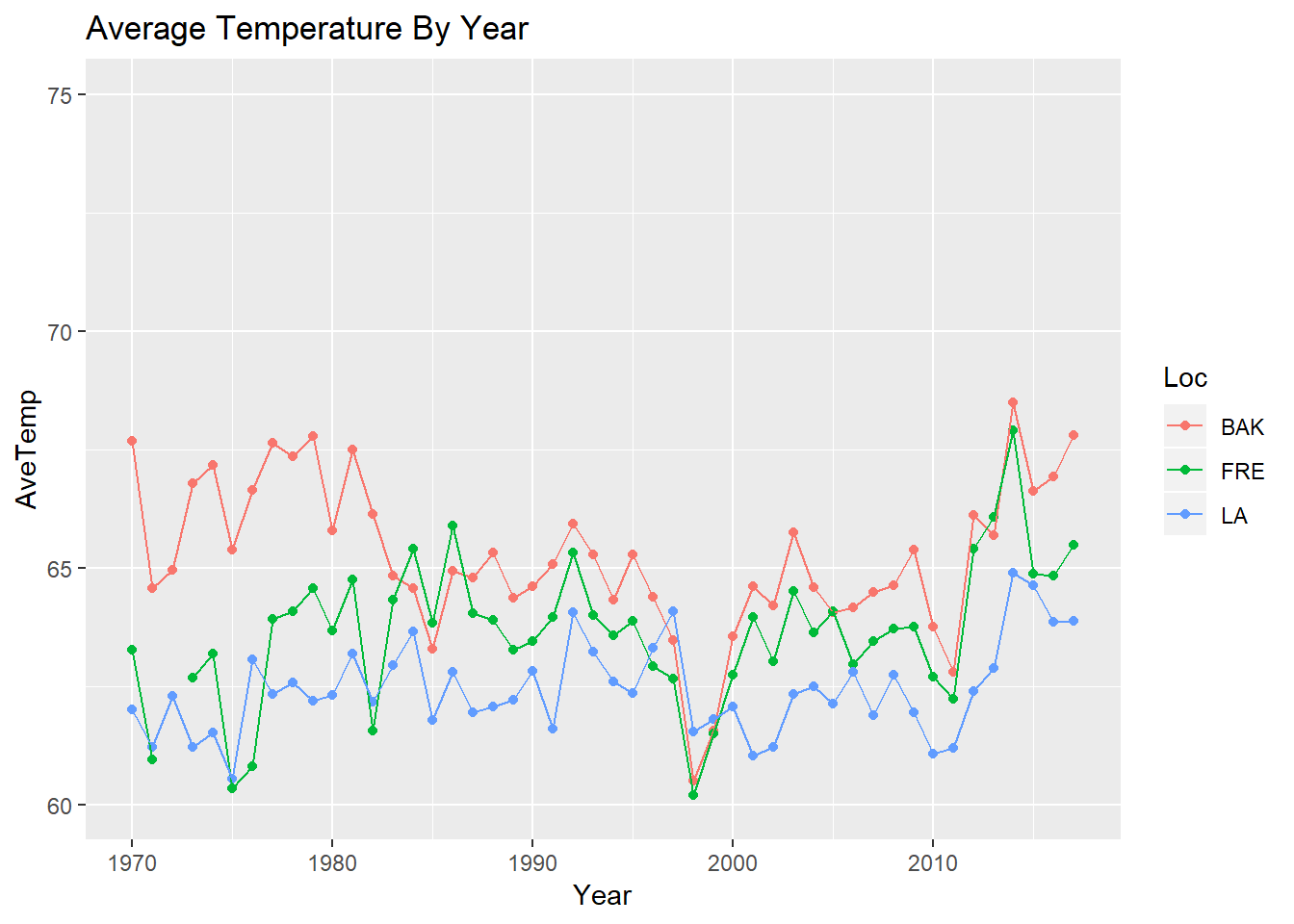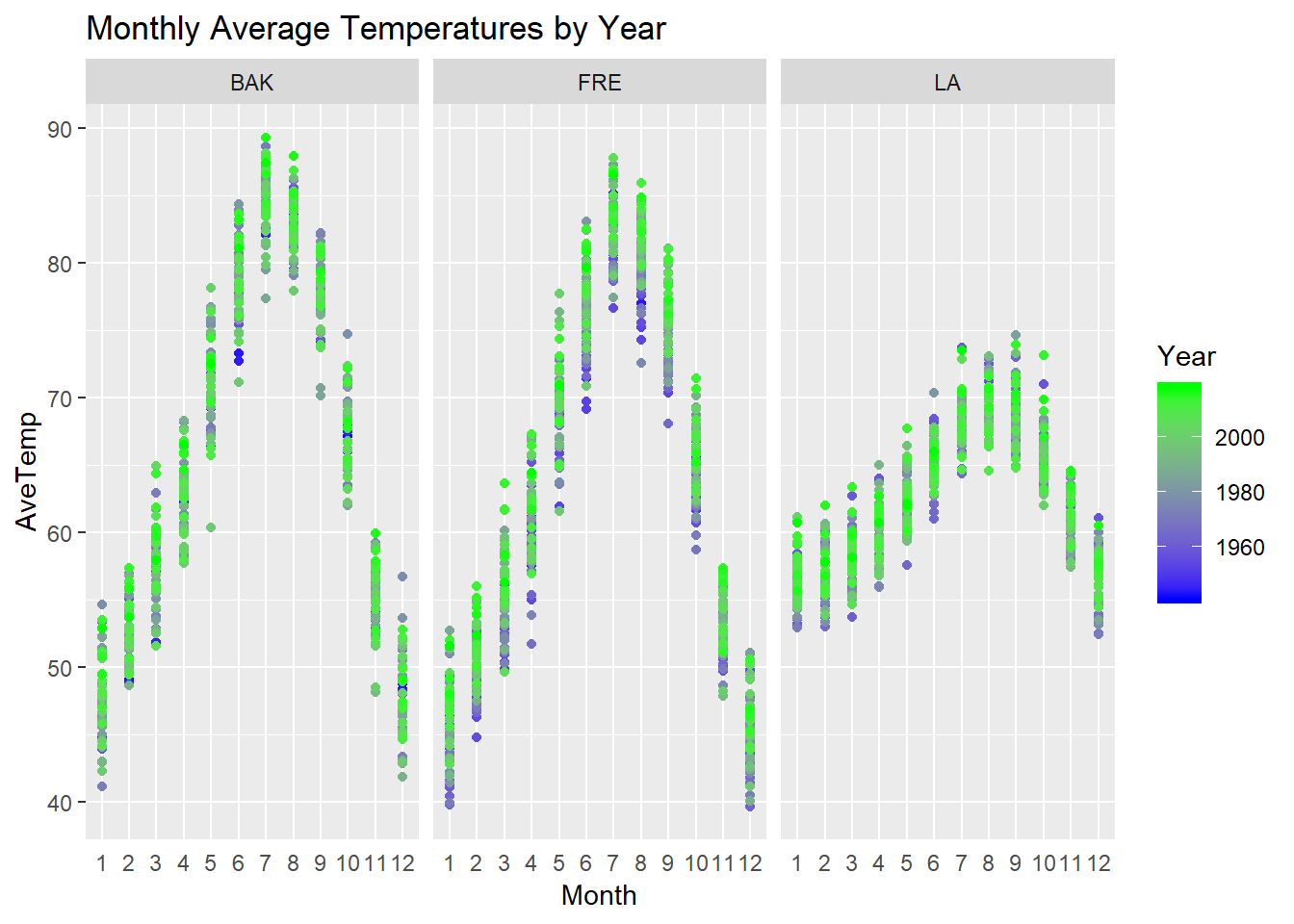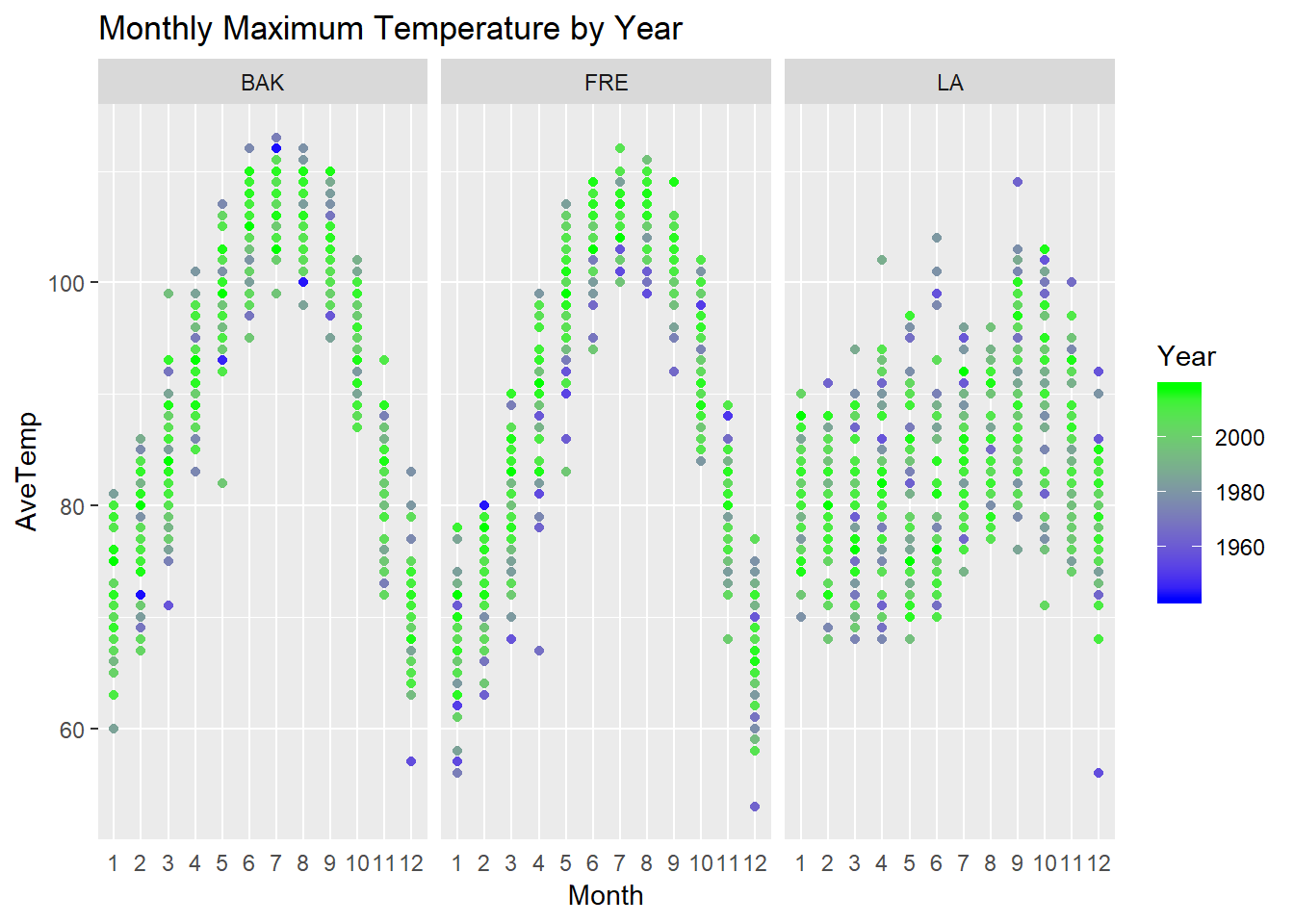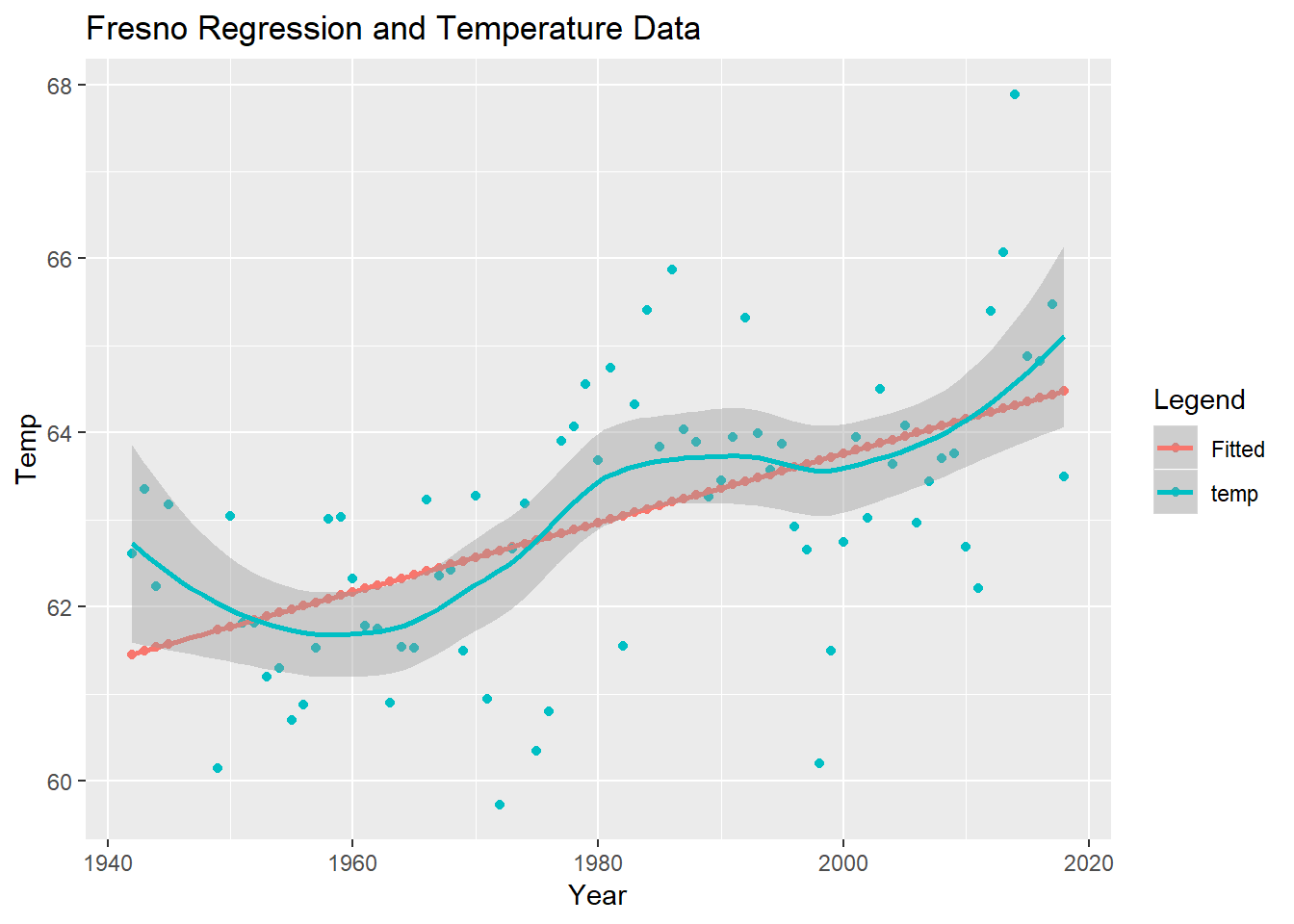This time on the Five Minute Analyst, we take a look at climate data from three cities in California, our home. A lot has been said about climate - and data in the popular pres - over the past few years. We decided to take a look at three California Cities - Bakersfield, Los Angeles, and Fresno - over a long time period. These cities were chosen because of their geographic diversity and length of record.

## Observations on the climate of California from Data

library(magrittr); library(dplyr);library(data.table); library(ggplot2); library(reshape2); library(lubridate)
F = fread("ClimDat.csv")
F$Date %<>% as.Date() F %>% group_by(Loc, Year) %>% summarize(AveTemp = mean(HOURLYDRYBULBTEMPF, na.rm = TRUE)) %>% ggplot(aes(x = Year, y = AveTemp, color = Loc)) + geom_point() + geom_line()+ xlim(c(1970, 2017)) + ylim(60, 75) + ggtitle("Average Temperature By Year")F$Month = month(F$Date) F$Month %<>% as.factor()

F %>% group_by(Month, Year, Loc) %>%  summarize(AveTemp = mean(HOURLYDRYBULBTEMPF, na.rm = TRUE)) %>% ggplot(aes(x = Month, y = AveTemp, color = Year )) + geom_point() + facet_wrap(~Loc) + theme(axis.ticks.x = element_blank()) + scale_color_gradient(low = 'blue', high = 'green') + ggtitle("Monthly Average Temperatures by Year")F %>% group_by(Month, Year, Loc) %>%  summarize(AveTemp = max(HOURLYDRYBULBTEMPF, na.rm = TRUE)) %>% ggplot(aes(x = Month, y = AveTemp, color = Year )) + geom_point() + facet_wrap(~Loc) + theme(axis.ticks.x = element_blank()) + scale_color_gradient(low = 'blue', high = 'green') + ggtitle("Monthly Maximum Temperature by Year")The first task here - as it is in most analyses, it to plot the data. It is particularity important in a task like this to keep an ‘open mind’ when looking at data; particularly - as we shall see below - the effects are small. Upon looking at this plot, there is not an obvious ‘smoking guns’ implying either the presence or absence of climate change. To do a more nuanced consideration, and look at this more precisely, we will perform a a standard linear regression of Average Temperature vs. year.

LMave = function(Dat, loc, FirstYear = 0){
library(magrittr); library(dplyr)
Dat %>% filter(Year > FirstYear) %>%  filter(Loc == loc) %>% mutate(Y2 = Year - min(Year)) %>% filter(Y2 > 0) %>% group_by(Y2) %>% summarize( temp = mean(HOURLYDRYBULBTEMPF, na.rm = TRUE), Year = max(Year)) -> zz

zz %>% lm(temp~ Y2, data = .) -> zzz

zz$Fitted = zzz$fitted.values
zz$Resid = zzz$residuals

return(list(AugDat = zz, Model = zzz))
}

LMmax = function(Dat, Loc, FirstYear = 0){
library(magrittr); library(dplyr)
Dat %>% filter(Year > FirstYear) %>%  filter(Loc == Loc) %>% mutate(Y2 = Year - min(Year)) %>% filter(Y2 > 0) %>% group_by(Y2) %>% summarize( maxtemp = max(HOURLYDRYBULBTEMPF, na.rm = TRUE), Year = max(Year)) -> zz

zz %>% lm(maxtemp~ Y2, data = .) -> zzz

zz$Fitted = zzz$fitted.values
zz$Resid = zzz$residuals

return(list(AugDat = zz, Model = zzz))
}
BAKave = LMave(F, "BAK")
LAave = LMave(F, "LA")
FREave = LMave(F, "FRE")

From these regressions, we see that there is only one case where the trend in temperature is an upwards ‘slam dunk’. In Fresno, the evidence that the temperature is rising at .039 degrees per year is pretty resounding (p value 3.9 x $$10^{-7}$$). Bakersfield’s regression shows a rate of .06 degrees per year (p-value of .027), which most practitioners still consider to be significant (against an $$\alpha$$ of .05) . In Los Angeles, there is not sufficient evidence (with a linear model) to support temperature rise with this data (p-value .15).

FREave\$AugDat %>% select(Year, Fitted, temp) %>% melt(id.vars = "Year") %>% rename("Temp" = "value", "Legend" = "variable") %>% ggplot(aes(x = Year, y = Temp, color = Legend)) + geom_point() + geom_smooth() + ggtitle("Fresno Regression and Temperature Data")# Conclusion

I hope that this little bit of data analysis will encourage our readers to think about this problem for themselves - specifically by obtaining their own data and repeating (or expanding upon) the work we do here. In the interests of scientific exploration, the code to this analysis is posted here. Given the upgrades in computing and availability of data, concerned citizens can simply do their own homework now and in the future.

### Acknoledgement

I would like to thank my intern, Jesse Ruediger, for drawing my attention to this problem, and also for collecting the data used in the analysis. I would also like to thank my colleague Dr. Cara Albright for introducing me to NOAA Data.